# The OEIS and the Recamán Sequence7

Posted by Cleve Moler,

Here are the first 12 integers from an infinite sequence defined by a deceptively simple rule. Can you see the pattern? Try to predict the next number in the sequence.

0, 1, 3, 6, 2, 7, 13, 20, 12, 21, 11, 22


### Contents

#### OEIS

The OEIS, the On-Line Encyclopedia of Integer Sequences, is a mathematical treasure chest. Established in 1964 by Neal J. A. Sloan, the OEIS has over 300,000 entries. Dozens are added each week. Anyone who registers can propose a new entry. A team of 130 volunteer editors then review the proposals and create new entries.

A powerful search engine accepts queries made from short subsequences and finds all the entries in the encyclopedia that contain that query. It's similar to a search engine that identifies a piece of music from a few notes.

Here is the link, https://oeis.org. One way to introduce yourself to the OEIS is to take a look at https://oeis.org/OEIS_pics.html. Or, watch the OEIS movie on YouTube, https://www.youtube.com/watch?v=LCWglXljevY.

#### A005132

Did you try to guess the next number is our sequence yet? You can ask the OEIS. If you enter the 12 numbers in the search window, you get something like this.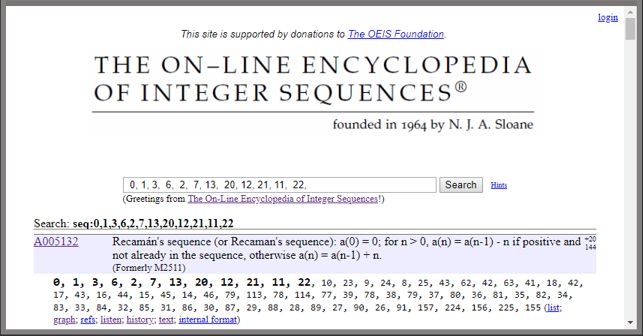So, you can see that the next number is 10.

Each entry in the encyclopedia has an identifier; for this sequence the identifier is A005132. We could also reach A005132 by searching for "A005132" or "Recaman".

Neal Sloan has said this is one of his favorite sequences in the entire collection. He learned about it in a 1991 letter from Columbian mathematician Bernardo Recamán Santos.

In the past few weeks I've become infatuated with Recamán sequence.

#### The rule

Starting with $a_0$ = 0, the sequence is generated by the recurrence

$$a_n = a_{n-1} \pm n$$

The minus sign is preferred. It is used if the resulting $a_n$ is positive and not already in the sequence; otherwise the plus sign is used.

So, for n = 1, 2, 3 the plus sign is required and the rule generates the familiar triangle numbers 1, 3, 6. But when n = 4 it is possible to use the minus sign because

$a_4$ = $a_3$ - 4 = 6 - 4 = 2

is not already in the sequence.

#### recaman.m

Here is my MATLAB function.

   type recaman

function R = recaman(m)
% R = recaman(n) is the first n terms of Recamán's sequence.
% OEIS A005132, https://oeis.org/A005132.
a = 0;
R = a;
for n = 1:m-1
if a > n && ~ismember(a-n,R)
a = a-n;
else
a = a+n;
end
R(n+1) = a;
end
end


It turns out to be faster for the sequence array R to grow during the recurrence than it is to preallocate it with R = zeros(1,m).

#### n = 12

Let's visualize the Recamán sequence. A plot made with semicircles connecting the terms is especially illuminating. The first small semicircle, on the left, has diameter one and end-points at the first two elements, 0 and 1. Subsequently, the diameter of the n-th semicircle is n and its end-points are $a_{n-1}$ and $a_n$. This plot ends on the right at $a_{12}$ = 22

I learned about this way of plotting the Recamán sequence from the YouTube channel Numberphile in a video featuring Alex Bellos and Edmund Harriss.

   set(gcf,'position',[200 200 480 360])
n = 12;
recaman_circles(n)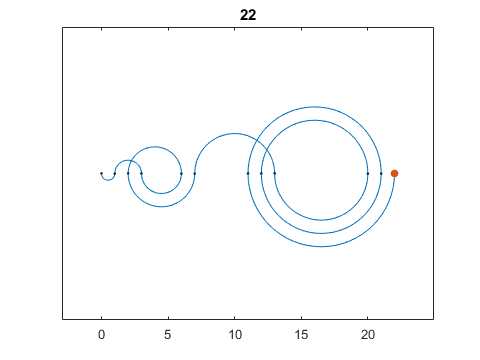A conventional MATLAB plot of the sequence versus index is also worth a look. The title is the last term, $a_n$.

   recaman_plot(n)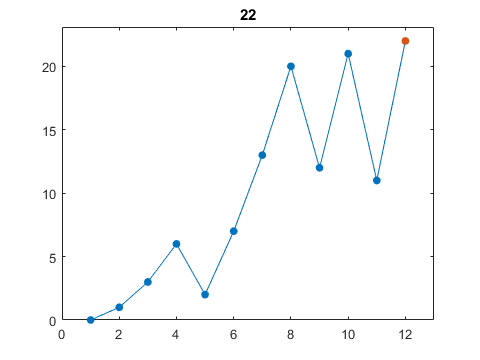#### n = 66

The value n = 66 is a good stopping point for the plots. The next value would jump from $a_{66}$ = 91 to $a_{67}$ = 157.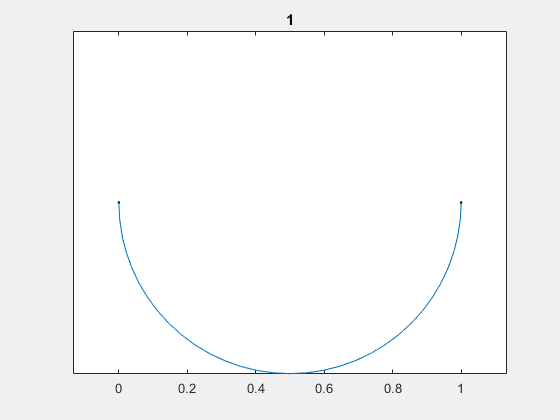The line plot is showing its characteristic behavior -- stretches of sawtooth oscillation with increasing amplitude separated by chaotic jumps to new "energy levels".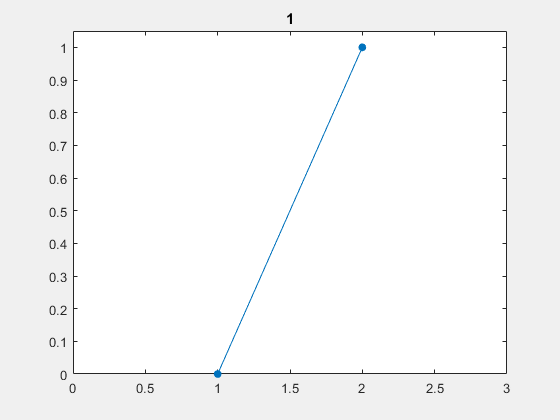#### n = 300

This behavior with 300 terms continues indefinitely.

   n = 300;
recaman_plot(n)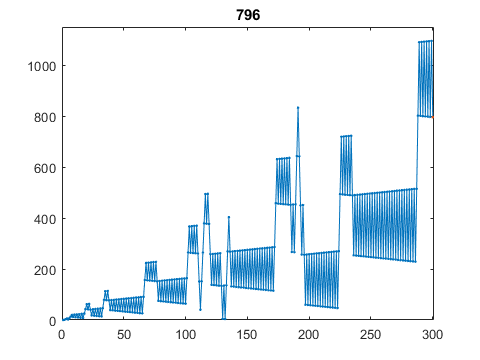#### Complexity

The computational complexity of recaman(n) is O(n^2). It takes about six minutes to generate a million terms on my laptop.

#### Every integer?

The Recamán recurrence is designed to hit every positive integer. Does it succeed? For each k > 0, is there a value of n for which $a_n$ = k. This is an open question. Sometimes n has to be surprisingly large.

Take k = 4.

   k = 4
R = recaman(1000);
n = find(k == R)

k =
4
n =
132


Take k = 19.

   k = 19
R = recaman(100000);
n = find(k == R)

k =
19
n =
99735


Take k = 852655. Wait, don't try that. Nobody knows if the sequence ever hits this value. Benjamin Chaffin has computed 10^230 terms without seeing 852655.

#### Music

I can't easily include audio in my blog. You'll have to do it yourself. For a real treat, go to https://oeis.org/A005132 and click on "listen".

#### Another sequence

Let's have a little more fun with the OEIS. There are only a handful of people in the world that know the next term in this sequence.

9, 6, 3, 9, 7, 2, 3, 8


To find out, enter these numbers in the search window at https://oeis.org. Or, bring up your MATLAB and

edit membrane


Get the MATLAB code

Published with MATLAB® R2018a

### Note

Sini replied on : 1 of 7
Very nice play. But, why is that: "It turns out to be faster for the sequence array R to grow during the recurrence than it is to preallocate it with R = zeros(1,m)." Matlab still hints me to consider preallocation. Indeed, *without* preallocation runs almost double the speed with m=1e5.
Cleve Moler replied on : 2 of 7
I'm afraid I don't know why. Can any reader help? See the next comment.
David Hruska replied on : 3 of 7
Neat stuff! Here's a version of the code that uses more memory (to maintain a logical array of visited numbers, thus avoiding the costly ismember call) to reduce the execution time:
function R = recaman2(m)
R = zeros(1,m);
a = 0;
R(1) = a;
for n = 1:m-1
if a > n && ~usedMask(a-n)
a = a-n;
else
a = a+n;
end
R(n+1) = a;
end
end

Here are the numbers for my machine:
>> tic; recaman(1e6); toc
Elapsed time is 131.435213 seconds.
>> tic; recaman2(1e6); toc
Elapsed time is 0.015423 seconds.

Cleve Moler replied on : 4 of 7
Thanks, David. Why is this so much faster?
Marcelo Avila replied on : 5 of 7
David, I saw your code and became intrigued with the line
if a > n && ~usedMask(a-n)
when n > a. But after some research I found the link about "Logical Operators: Short-Circuit && ||" (Logical operations with short-circuiting). So now I understand what is going on in that "if" statement. Very interesting implementation.
David Hruska replied on : 6 of 7
The bottleneck in the original code is the ISMEMBER function. While a single call is pretty fast, the time adds up when called inside a for-loop. Furthermore, its run-time is roughly proportional to the number of elements that need to be searched through:
>> tic; ismember(0,1:1e6); toc
Elapsed time is 0.008192 seconds.
>> tic; ismember(0,1:1e7); toc
Elapsed time is 0.076229 seconds.
>> tic; ismember(0,1:1e8); toc
Elapsed time is 0.776335 seconds.

Thus, repeating the ISMEMBER call M times on an array that grows from 1 to M elements gives rise to the O(n^2) behavior. When preallocation is used, each of the M iterations through the for-loop calls ISMEMBER on an array with M elements. This is still O(n^2) but with a larger constant. Growing the array inside the for-loop is not a significant contributor to the run-time when compared with ISMEMBER. My version of the code uses some additional memory to store a logical array to keep track of numbers that have been "used". By avoiding the ISMEMBER call, it runs faster.
Andy Stamps replied on : 7 of 7
To build on David's reply, ISMEMBER will necessarily be at least O(n) and if sorting is being done internally then at least O(n*log(n)). The logical test in David's RECAMAN2 accesses a single element of an array, and therefore retains O(1) complexity. Hence, RECAMAN2 is overall O(n) while RECAMAN is O(n^2) or worse.